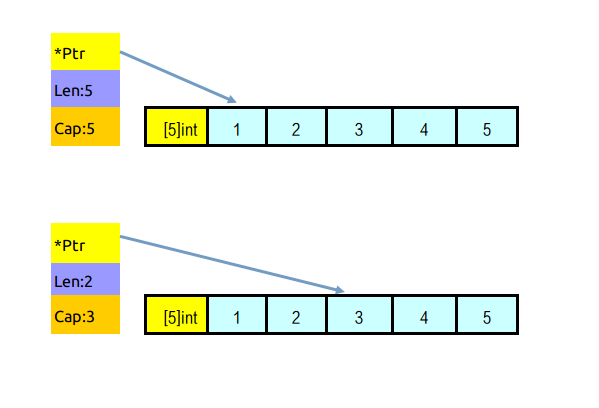func main() {
arr1 := int{1, 2, 3, 4, 5}
slice1 := arr1[1:2]
slice1 = append(slice1, 6, 7, 8)
fmt.Println("slice1:", slice1)
fmt.Println("arr1:", arr1)

arr2 := int{1, 2, 3, 4, 5}
slice2 := arr2[1:3]
slice2 = append(slice2, 6, 7, 8)
fmt.Println("slice2:", slice2)
fmt.Println("arr2:", arr2)
}


\$ go run sliceTrap.go
slice1: [2 6 7 8]
arr1: [1 2 6 7 8] //神奇地，原数组被改变了
slice2: [2 3 6 7 8]
arr2: [1 2 3 4 5] //一切正常


type slice struct{
Ptr *int //指向分配的数组的指针
Len int  // 长度
Cap int  // 容量
}


func make([]T, len, [cap]) []T


slice：= make([]int, 5, 5)
//注意：Go的默认零值
// slice == []int{0, 0, 0, 0, 0}


slice:=make([]int,5)
//len(slice)==5
//cap(slice)==5


arr := int{1, 2, 3, 4, 5}
s1 := arr[1:4]//对数组进行切片
//len(s1)== 3 //len为切片开始位置到结束位置的个数
//cap(s1)=4 //容量为原数组总长度减开始位置

s2:=s1[2:] //对slice进行切片
//len(s2)==1 //len为切片开始位置到结束位置的个数
//cap(s2)==2 //容量为原slice总容量减开始位置


slice := []int{1, 2, 3, 4, 5}
newslice:=slice[2:4]slice的容量值，限定了slice可容纳元素的最多个数，当我们往slice里添加新元素，导致元素个数超过容量时（len>cap）,则需要对slice进行扩容（Growing slices）。append方法的调用就是典型的扩容示例。我们来来模拟一下append方法的基本实现：

func AppendInt(slice []int, data ...int) []int {
m := len(slice)
n := m + len(data)
if n > cap(slice) {//判断是否需要扩容
//创建新的slice，其实也就是开辟了一个新的内存空间，
//并返回了指向新地址的指针（一般会是增加为总需要长度的两倍，加1是为了防止n=0的情况）
newSlice := make([]int, (n+1)*2)

//将旧的slice的元素值，copy到新创建的slice
copy(newSlice, slice)

//关键的一步，slice重新指向新分配的slice，这也就是本文开头的例子里arr2的值没有变化的原因
slice = newSlice
}
slice = slice[0:n]
//由于本步的copy操作，直接改变了原slice(如果没有重分配的话)里元素的值，
//所以导致了本文开头的例子里arr2的值的变化
copy(slice[m:n], data)
return slice
}


1. 创建一个容量更大的slice（扩容）。与对slice进行切片操作不同，这个slice是全新的，它的数组也是全新的，指针也是指向新数组的首位置。

2. 新slice创建好后，会将原来被append的slice的元素内容进行值复制到新的slice。

3. 将要被append元素，追加到新slice的末尾。

void runtime·appendslice(SliceType *t, Slice x, Slice y, Slice ret)
{
intgo m;
uintptr w;
void *pc;
uint8 *p, *q;

m = x.len+y.len;
w = t->elem->size;

if(m < x.len)
runtime·throw("append: slice overflow");

if(m > x.cap)
growslice1(t, x, m, &ret);
else
ret = x;

if(raceenabled) {
// Don't mark read/writes on the newly allocated slice.
pc = runtime·getcallerpc(&t);
if(m > x.cap)
// write x[len(x):len(x)+len(y)]
if(m <= x.cap)
runtime·racewriterangepc(ret.array+ret.len*w, y.len*w, w, pc, runtime·appendslice);
}

// A very common case is appending bytes. Small appends can avoid the overhead of memmove.
// We can generalize a bit here, and just pick small-sized appends.
p = ret.array+ret.len*w;
q = y.array;
w *= y.len;
if(w <= appendCrossover) {
if(p <= q || w <= p-q) // No overlap.
while(w-- > 0)
*p++ = *q++;
else {
p += w;
q += w;
while(w-- > 0)
*--p = *--q;
}
} else {
runtime·memmove(p, q, w);
}
ret.len += y.len;
FLUSH(&ret);
}

static void growslice1(SliceType *t, Slice x, intgo newcap, Slice *ret)
{
intgo m;

m = x.cap;

// Using newcap directly for m+m < newcap handles
// both the case where m == 0 and also the case where
// m+m/4 wraps around, in which case the loop
// below might never terminate.
if(m+m < newcap)
m = newcap;
else {
do {
if(x.len < 1024)
m += m;
else
m += m/4;
} while(m < newcap);
}
makeslice1(t, x.len, m, ret);
runtime·memmove(ret->array, x.array, ret->len * t->elem->size);
}